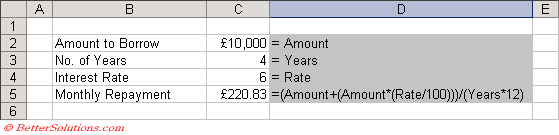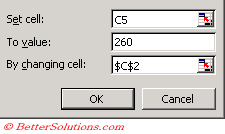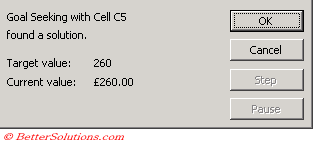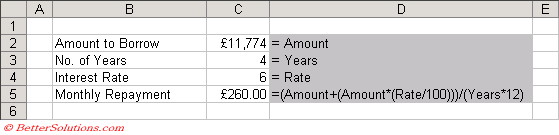# Loan Repayment

Lets consider a simple loan repayment.
If we borrow £10,000 with an interest rate of 6.0% per annum and the loan has to be repaid over 4 years we can use a simple formula to calculate the monthly repayment.
What if we wanted to know how much we could borrow assuming a given monthly repayment of £260.00.

• We could either do this using trial and error entering different borrow amounts until we are close although this approach is not very practical.

• We could change the formula to calculate the "Amount to Borrow" but this will be time consuming.

• You can use the Goal Seek to perform a "what if" analysis. We can use our original formula and let the Goal Seek iteration process perform the trial and error for us.

### Create the Table

Create the following table and the following named ranges:
"C2" = Amount
"C3" = Years
"C4" = RateA formula is entered into cell "C5" that will calculate the monthly repayment based on the other 3 values.
Given the values above we can see that the monthly repayment would be £220.83.
Lets suppose though that we had not decided on the exact amount to borrow or even the number of years over which we wanted to pay off the loan.
In this case we could change the "Amount to Borrow" to say £11,000 and the "Monthly Repayment" would change automatically to £242.92.

### Using Goal Seek

Select cell "C4" (i.e. the cell containing the formula) and press (Tools > Goal Seek).
The "Set cell" is the cell that contains the formula.
The "To value" is the value that you want to find a solution for.
The "By changing cell" is the cell containing the value that you want to change in order to change the formula (in the "Set cell").Press OK to perform an iterative technique to try and find the specified goal.
Different values will be tried repeatedly for the value in cell "C2" until the value in cell "C5" is £260.00.
This simple example will be solved very quickly and a confirmation dialog box will be displayed.Pressing OK will transfer the correct solution to the cells and the corresponding amount to borrow will be £11,774.You can press Cancel to not overwrite the results.

### Important

You can use Goal Seek with complex financial models as well as simple problems. Link the final result to the other variable cells to drive the model changes.